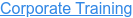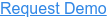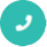#### 15+ Categories. 500+ IT Courses to Learn.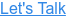by Sachin, on Jul 20, 2022 8:05:36 PM## Ans

TensorFlow is a Python-based library which is used for creating machine learning applications. It is a low-level toolkit to perform complex mathematics. It offers users the customizability option to build experimental learning architectures. It also helps the users to work with them, and to turn them into running software. It was initially created by researchers and engineers working on the Google Brain Team, and It became open source in 2015.

TensorFlow is made up of two words Tensor and Flow; a tensor is known as data representation for multi-dimensional array and flow means a series of operations performed on tensors.

## Ans

There are three types of Tensors used to create neural network models:

• Constant Tensor
Constant Tensors are used as constants, as the name suggests. They create a node that takes a value and does not change it. A constant can be created using tf.constant.
tf.constant(value, dtype=None, shape=None, name='Const', verify_shape=False)
It accepts the five arguments.
• Variable Tensor
Variable Tensors are the nodes which provide their current value as output. It means that they can retain their value over multiple executions of a graph.
• Place Holder Tensor
Placeholders Tensors are essential than variables. These are used to assign data in a later time. Placeholders are the nodes whose value is fed at the time of execution. Assume, we have inputs to our network which are dependent on some external data. Also, we do not want our graph to depend on any real value while developing the graph, then Placeholders are useful datatype. We can even build a graph without any data.

## Ans

TensorBoard is a suite of visualizing tools for inspecting and understanding TensorFlow runs and graphs. It is an easy solution to Tensorflow offered by the creators that let us visualize the graphs. It plots quantitative metrics about the graph with additional data like images to pass through it.

## Ans

Tensorflow has APIs support for a wide variety of languages such as Matlab and C++. Researchers are continuously trying to making it better. A javascript library, tensorflow.js, has also been introduced for training and deploying machine learning models.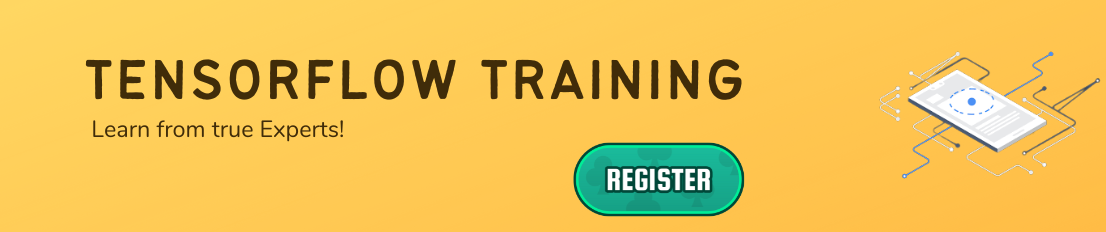## Ans

• It can be easily trained on CPU as well as GPU for distributed computing.
• It has auto differentiation capabilities.
• It has platform flexibility.
• It is easily customizable and open-source.

## Ans

• It does not provide support for OpenCL (Open Computing Language).
• It requires prior knowledge of advanced calculus and linear algebra along with a pretty good understanding of Machine learning.
• It has GPU memory conflicts with Theano if imported in the same scope.

## Ans

TensorFlow allows the formulation of graphs for the models, which is the collection of tensors and nodes.

TensorBoard helps to perform the visualization through the graphs. TensorBoard is a collection of web services that help in debugging, eager execution, and a dynamic runtime. It’s a tool that eases the debugging with its functionality and is the sole method of visualization.

## Ans

The computation of the difference between the predicted and actual value using a function is known as the loss function. The value of this function defines the amount of difference in values.

Hence at each run, the Gradient Function optimizer checks for the changes which can help to improve the model. With the help of optimizer, the loss reduces to the minimum and attains maximum accuracy.

## Ans

 CNN RNN Convolutional Neural Network Recurrent Neural Network Known as the feed-forward model For the series of data Memoryless model Requires memory to store previous inputs Cannot handle sequential data Can handle Sequential data Used for Image recognition Used for Text recognition Can handle fixed length of input/ output Can handle arbitrary lengths of input/ output Feature compatibility is more Feature compatibility is less Handles permanent data Handles temporary data

## Ans

• Linear regression
• Linear classification
• Boosted tree classification
• Boosted tree regression
• Deep learning classification

## Ans

Neural networks as the name suggests are a network of elemental processing entities that together make a complex form. There can be Artificial Neural Networks and Biological Neural Networks. The use of artificial neural networks is more common as they try to imitate the mechanics of the human brain.

## Ans

• TFLearn: A popular Python package
• TensorLayer: For layering architecture support
• Pretty Tensor: Google’s project providing a chaining interface
• Sonnet: Provides a modular approach to programming

## Ans

TensorFlow contains certain libraries used for abstraction such as Keras and TF-Slim. They are used to provide high-level access to data and model life cycle for programmers using TensorFlow. This can help them easily maintain clean code and also reduce the length of the code exponentially.

## Ans

A graph explorer is used to visualize a graph on TensorBoard. It is also used for the inspection operations of a model in TensorFlow. To easily understand the flow in a graph, it is recommended to use a graph visualizer in TensorBoard.

## Ans

• Scalar dashboard
• Image dashboard
• Graph dashboard
• Text dashboard
• Distributer dashboard
• Histogram dashboard

## Ans

 TensorFlow PyTorch Developed by Google Developed by Facebook No support for runtime graph operations Provides computational graph operations at runtime Offers TensorBoard for visualization No visualization tools offered in the bundle Based on the Theano library Based on the Torch library

## Ans

Deep Speech is a speech-to-text engine that is open-source and uses TensorFlow. It is trained based on Machine Learning techniques and uses a simple syntax to process speech from an input to produce textual output on the other end.

## Ans

TensorFlow JS is a library that gives users the functionality of using browsers to run Machine Learning models. High-level APIs work with JavaScript to support a variety of entities in the backend, such as WebGL, to use a GPU to render functionality (if available). Models can be imported, re-trained, and executed easily by just using a browser.

## Ans

Activation functions are functions applied to the output side of a neural network that serves to be the input of the next layer. It forms a very important part of neural networks as it provides nonlinearity that sets apart a neural network from logistic regression.

## Ans

The process of handling the complexity that follows when optimizing inferences can be greatly minimized using TensorFlow. Model quantization is primarily used to reduce the representation of weights and also for the storage and computation of the activation function.

Using model quantization provides users with two main advantages:

• Support for a variety of CPU platforms
• SIMD instruction handling capabilities

• Momentum
• RMSprop

## Ans

Numerical loss functions:

• L1 loss
• L2 loss
• Pseudo-Huber loss

Categorical loss functions:

• Hinge loss
• Cross-entropy loss
• Sigmoid-entropy loss
• Weighted cross-entropy loss

Each of the loss functions mentioned above has a specific use based on the input data and the type of modeling involved.